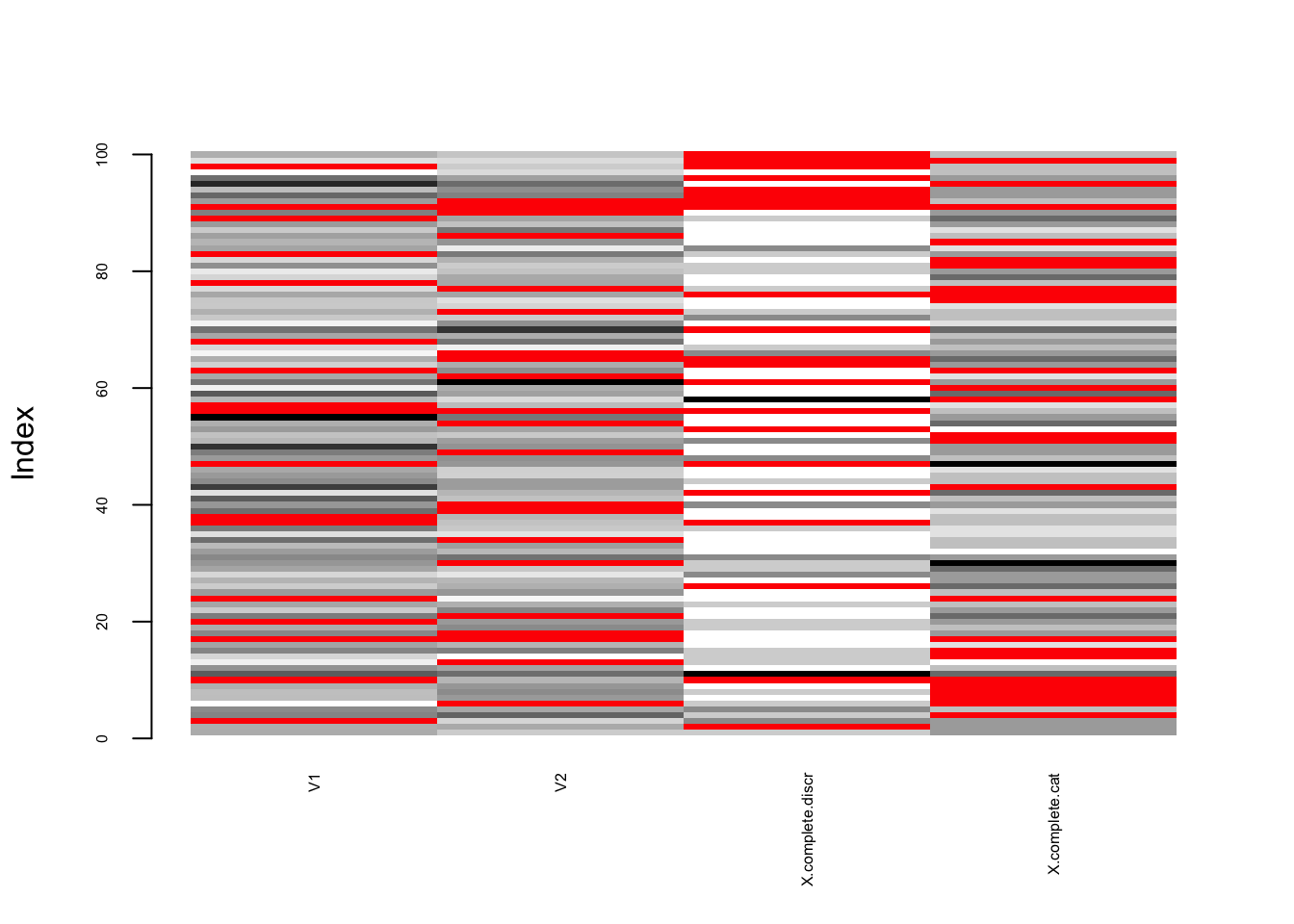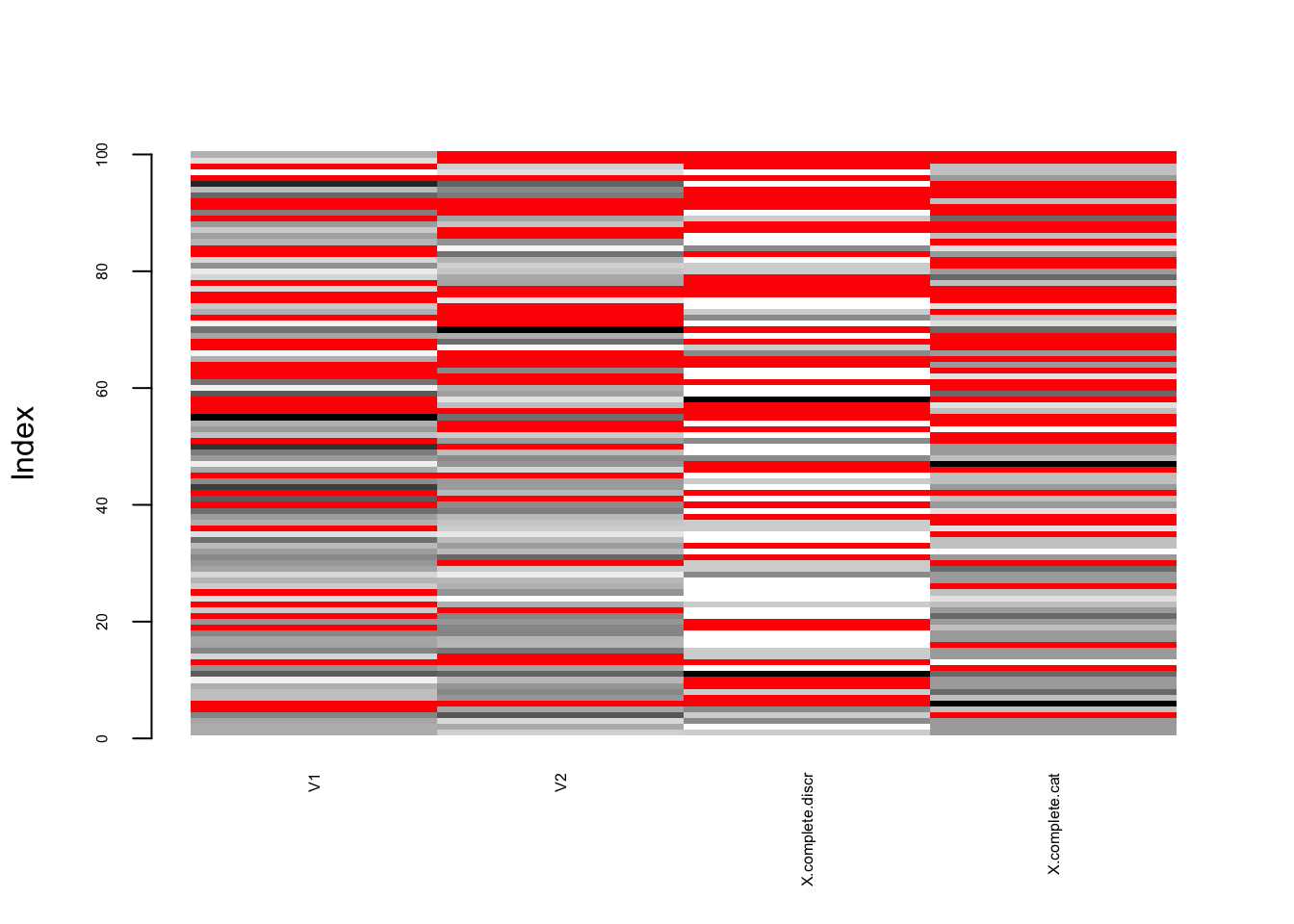Missing values occur in many domains and most datasets contain missing values (due to non-responses, lost records, machine failures, dataset fusions, etc.). These missing values have to be considered before or during analyses of these datasets.

Now, if you have a method that deals with missing values, for instance imputation or estimation with missing values, how can you assess the performance of your method on a given dataset? If the data already contains missing values, than this does not help you since you generally do not have a ground truth for these missing values. So you will have to simulate missing values, i.e. you remove values – which you therefore know to be the ground truth – to generate missing values.

The mechanisms generating missing values can be various but usually they are classified into three main categories defined by (Rubin 1976): missing completely at random (MCAR), missing at random (MAR) and missing not at random (MNAR). The first two are also qualified as ignorable missing values mechanisms, for instance in likelihood-based approaches to handle missing values, whereas the MNAR mechanism generates nonignorable missing values. In the following we will briefly introduce each mechanism (with the definitions used widely in the literature) and propose ways of simulations missing values under these three mechanism assumptions. For more precise definitions we refer to references in the bibliography on the R-miss-tastic website.

# 1 Notations

Let’s denote by $$\mathbf{X}\in\mathcal{X_1}\times\dots\times\mathcal{X_p}$$ the complete observations. We assume that $$\mathbf{X}$$ is a concatenation of $$p$$ columns $$X_j\in\mathcal{X_j}$$, $$j\in\{1,\dots,p\}$$, where $$dim(\mathcal{X_j})=n$$ for all $$j$$.

The data can be composed of quantitative and/or qualitative values, hence $$\mathcal{X_j}$$ can be $$\mathbb{R}^n$$, $$\mathbb{Z}^n$$ or more generally $$\mathcal{S}^n$$ for any discrete set $$S$$.

Missing values are indicated as NA (not available) and we define an indicator matrix $$\mathbf{R}\in\{0,1\}^{n\times p}$$ such that $$R_{ij}=1$$ if $$X_{ij}$$ is observed and $$R_{ij}=0$$ otherwise. We call this matrix $$\mathbf{R}$$ the response (or missingness) pattern of the observations $$\mathbf{X}$$. According to this pattern, we can partition the observations $$\mathbf{X}$$ into observed and missing: $$\mathbf{X} = (\mathbf{X}^{obs}, \mathbf{X}^{mis})$$.

We generate a small example of observations $$\mathbf{X}$$:

suppressPackageStartupMessages(require(MASS))
suppressPackageStartupMessages(require(norm))
suppressPackageStartupMessages(require(VIM))
suppressPackageStartupMessages(require(ggplot2))
suppressPackageStartupMessages(require(naniar))

source("amputation.R")
set.seed(1)
# Sample data generation ------------------------------------------------------
# Generate complete data
mu.X <- c(1, 1)
Sigma.X <- matrix(c(1, 1, 1, 4), nrow = 2)
n <- 100
X.complete.cont <- mvrnorm(n, mu.X, Sigma.X)

lambda <- 0.5
X.complete.discr <- rpois(n, lambda)

n.cat <- 5
X.complete.cat <- rbinom(n, size=5, prob = 0.5)

X.complete <- data.frame(cbind(X.complete.cont, X.complete.discr, X.complete.cat))
X.complete[,4] <- as.factor(X.complete[,4])
levels(X.complete[,4]) <- c("F", "E", "D", "C", "B", "A")

# 2 What data can be handled

With the main function produce_NA it is possible to generate missing values for quantitative, categorical or mixed data, provided that it is available in form of a data.frame or matrix.

Missing values can be generated following one or more of the three main missing values mechanisms (see below for details).

If the data is already incomplete, it is possible to add a specific amount of additional missing values, in the already incomplete features or other complete features.

Important: Currently there is no option available for the mains function produce_NA to specify that every observation must contain at least one value after amputation. Hence, in the data.frame output by produce_NA there might be empty observations.

Except for the MCAR mechanism, our function produce_NA internally calls the ampute function of the mice R-package. See (Schouten, Lugtig, and Vink 2018) for a detailed description of this latter function.

# 3 Use of produce_NA with default settings

## 3.1 Minimal set of arguments

In order to generate missing values for given data, produce_NA requires the following arguments:

• data: the initial data (can be complete or incomplete) as a matrix or data.frame
• mechanism: one of “MCAR”, “MAR”, “MNAR” (default: “MCAR”)
• perc.missing: the proportion of new missing values among the initially observed values (default: 0.5)

## 3.2 Value

produce_NA returns a list containing three elements:

• data.init: the initial data
• data.incomp: the data with the newly generated missing values (and the initial missing values if applicable)
• idx_newNA: a matrix indexing only the newly generated missing values

## 3.3 Example

On complete data

# Minimal example for generating missing data ------------------------
X.miss <- produce_NA(X.complete, mechanism="MCAR", perc.missing = 0.2)

X.mcar <- X.miss$data.incomp R.mcar <- X.miss$idx_newNA

writeLines(paste0("Percentage of newly generated missing values: ", 100*sum(R.mcar)/prod(dim(R.mcar)), " %"))
## Percentage of newly generated missing values: 21 %
matrixplot(X.mcar, cex.axis = 0.5, interactive = F)On incomplete data:

# Minimal example for generating missing data on an incomplete data set ------------------------
X.miss <- produce_NA(rbind(X.complete[1:50,], X.mcar[51:100,]), mechanism="MCAR", perc.missing = 0.2)

X.mcar <- X.miss$data.incomp R.mcar <- X.miss$idx_newNA

writeLines(paste0("Percentage of newly generated missing values: ", 100*sum(R.mcar)/prod(dim(R.mcar)), " %"))
## Percentage of newly generated missing values: 22 %
matrixplot(X.mcar, cex.axis = 0.5, interactive = F)# 4 Details on all available specifications

The main function produce_NA allows generating missing values in various ways. These can be specified through different arguments:

produce_NA(data, mechanism = "MCAR", perc.missing = 0.5, self.mask=NULL, idx.incomplete = NULL, idx.covariates = NULL, weights.covariates = NULL, by.patterns = FALSE, patterns = NULL, freq.patterns = NULL, weights.patterns = NULL, use.all=FALSE, logit.model = "RIGHT", seed = NULL)

## 4.1 Mechanisms

In order to define the different missing values mechanisms, both $$\mathbf{X}$$ and $$\mathbf{R}$$ are modeled as random variables with probability distributions $$\mathbb{P}_X$$ and $$\mathbb{P}_R$$ respectively. We parametrize the missingness distribution $$\mathbb{P}_R$$ by a parameter $$\phi$$.

### 4.1.1 MCAR

#### 4.1.1.1 Definition

The observations are said to be Missing Completely At Random (MCAR) if the probability that an observation is missing is independent of the variables and observations: the probability that an observation is missing does not depend on $$(\mathbf{X}^{obs},\mathbf{X}^{mis})$$. Formally this is: $\mathbb{P}_R(R\,|\, X^{obs}, X^{mis}; \phi) = \mathbb{P}_R(R) \qquad \forall \, \phi.$ #### Example

# Sample mcar missing data -----------------------------------------
mcar <- produce_NA(X.complete, mechanism="MCAR", perc.missing = 0.2)

X.mcar <- mcar$data.incomp R.mcar <- mcar$idx_newNA

writeLines(paste0("Percentage of newly generated missing values: ", 100*sum(R.mcar)/prod(dim(R.mcar)), " %"))
## Percentage of newly generated missing values: 22.75 %
matrixplot(X.mcar,  cex.axis = 0.5, interactive = F)